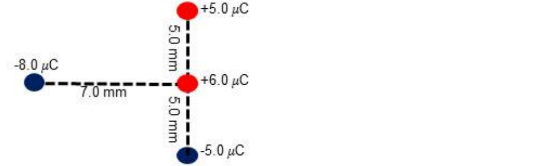Problem: Four charges are arranged as shown in the figure below each with a positive (red) charge or a negative (blue) charge. Find the net total force (magnitude and direction) exerted on the charge with + 6.0 micro coulomb by the other three charges.

FREE Expert Solution
96% (281 ratings)
Problem Details

Four charges are arranged as shown in the figure below each with a positive (red) charge or a negative (blue) charge. Find the net total force (magnitude and direction) exerted on the charge with + 6.0 micro coulomb by the other three charges.